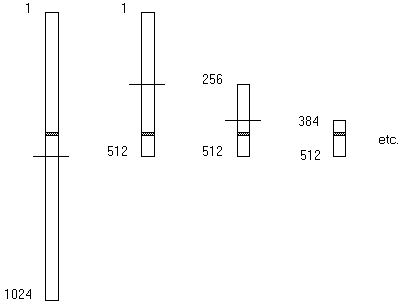Computer Science - C++ Lesson 26:  Searches:  Sequential & Binary

INTRODUCTION:               Searching for an item is a very important algorithm to a computer scientist.  What makes computers tremendously valuable is their ability to store and search for information.  For example, the search engines used to "surf" the Internet process incredible amounts of information.  Most word processors have spell-checking abilities, which require massive amounts of searching.  In this lesson, you will learn about a simple sequential search and the very effective binary search.

The key topics for this lesson are:

A.  Sequential Search

B.   Binary Search

C.  Recursive vs. Non-recursive Algorithms

VOCABULARY:                   SEQUENTIAL SEARCH           BINARY SEARCH

DISCUSSION:

A.  Sequential Search

1.   Searching a linear data structure such as an array can be as simple as checking through every value until you find what you are looking for.  A sequential search involves starting at the beginning of a list, sorted or not, and searching one-by-one.

2.   This unsophisticated approach is appropriate for small lists or unordered lists.

3.   The order of a sequential search is linear, O(N).

B.   Binary Search

1.   The word binary refers to the concept of two.

2.   Assuming that a list was previously sorted, a value is searched for by repeating the following steps:

a.   Divide the list in half

b.   Check to see if the value in the middle of the list equals the value being searched for.

c.   If the value is not found, then apply steps a, b, & c to the appropriate sublist.

3.   As an example, suppose we have a list of 1,024 sorted values and we search for a value that happens to be stored in position 492.  The list of 1,024 is split in half; the value is not found in position 512, so we proceed to search the top half.  Within the sublist from 1..512, we do another binary search sequence:  split; check; and binary search again.4.   The speed of binary search comes from the elimination of half of the data set each time.  The worst case scenario of searching a list of 1,024 elements are lists of size:

1024 --> 512 --> 256 --> 128 --> 64 --> 32 --> 16 --> 8 --> 4 --> 2 --> 1

If each arrow represents one binary search process, only ten steps are required to search a list of 1,024 numbers.  The answer to log21024 is 10.  If the size of the list doubled to 2,048, only one more step would be required in a binary search.

The efficiency of binary search is illustrated in this comparison of the number of entries in a list and the number of binary divisions required:

Number of Entries                     Number of Binary Divisions

1,024                                            10

2,048                                            11

4,096                                            12

...                                             ...

32,768                                            15

...                                             ...

1,048,576                                            20

N                                       log2N

5.   The order of binary search is O(log2N).

C.  Recursive vs. Non-recursive Algorithms

1.   The binary search algorithm can be coded recursively or non-recursively.  Here are some arguments for each method.

2.   A non-recursive version requires less memory and fewer steps by avoiding the overhead of making recursive calls.

3.   However, the recursive version is somewhat easier to understand and code.  The lab assignment can be coded as either  a recursive or non-recursive version of binary search.

SUMMARY/REVIEW:         Searching algorithms are widely used in programs.  Binary search is considered the best, assuming the list is sorted.

ASSIGNMENT: